# Step 3 - Orient last layer - OLL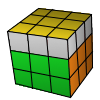While solving the Rubik's Cube with the advanced Fridrich method, when the first two layers (F2L) are solved we need to orient the last layer (OLL) so the upper face of the Rubik's Cube is all yellow. We don't care if the side colors don't match, we are going to permute the last layer (PLL) later. Here are a few animated examples. Press the play to start the animation.

You'll need to learn all the 57 algorithms below to complete this in one step. If this seems too many I recommend you learn the 2look OLL which uses only 9 algorithms but of course it's slower.

Let's group them according to their look.

### Dot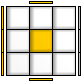R U B' l U l2' x' U' R' F R F'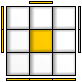R' F R F' U2 R' F R y' R2 U2 R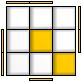y L' R2 B R' B L U2' L' B M'R' U2 x R' U R U' y R' U' R' U R' F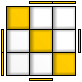(R U R' U) R' F R F' U2 R' F R F'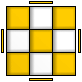M' U2 M U2 M' U M U2 M' U2 M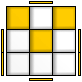R' U2 F (R U R' U') y' R2 U2 x' R U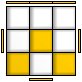F (R U R' U) y' R' U2 (R' F R F')

### Line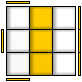R' U' y L' U L' y' L F L' F R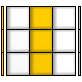R U' y R2 D R' U2 R D' R2 d R'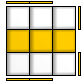F U R U' R' U R U' R' F'L' B' L U' R' U R U' R' U R L' B L

### CrossL U' R' U L' U (R U R' U) R(R U R' U) R U' R' U R U2 R'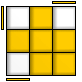L' U R U' L U R'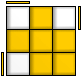R' U2 (R U R' U) R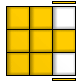R' F' L F R F' L' F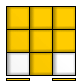R2 D R' U2 R D' R' U2 R'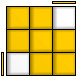R' F' L' F R F' L F

### 4 corners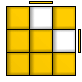M' U' M U2' M' U' ML' (R U R' U') L R' F R F'

### Shape _|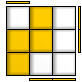L F R' F R F2 L'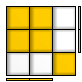F R' F' R U R U' R'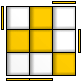R' U' R y' x' R U' R' F R U R'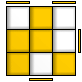U' R U2' R' U' R U' R2 y' R' U' R U B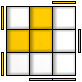F (R U R' U') (R U R' U') F'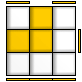L F' L' F U2 L2 y' L F L' F

### Shape |_U' R' U2 (R U R' U) R2 y (R U R' U') F'r U2 R' U' R U' r'R' U2 l R U' R' U l' U2 RF' L' U' L U L' U' L U FR' F R' F' R2 U2 x' U' R U R'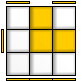R' F R F' U2 R2 y R' F' R F'

### Shape ¯|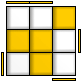R U R' y R' F R U' R' F' R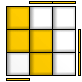L' B' L U' R' U R L' B L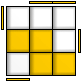U2 r R2' U' R U' R' U2 R U' M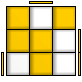x' U' R U' R2' F x (R U R' U') R B2

### Shape |¯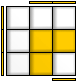L U' y' R' U2' R' U R U' R U2 R d' L'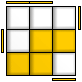U2 l' L2 U L' U L U2 L' U M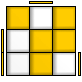R2' U R' B' R U' R2' U l U l'r' U2 (R U R' U) r

### C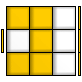R U x' R U' R' U x U' R'(R U R' U') x D' R' U R E'

### L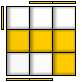R' F R U R' F' R y L U' L'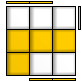L F' L' U' L F L' y' R' U RL' B' L R' U' R U L' B LR B R' L U L' U' R B' R'

### P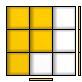F U R U' R' F'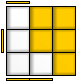R' d' L d R U' R' F' R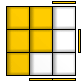L d R' d' L' U L F L'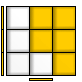F' U' L' U L F

### T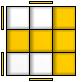F (R U R' U') F'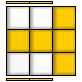(R U R' U') R' F R F'

### W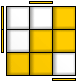L U L' U L U' L' U' y2' R' F R F'R' U' R U' R' U R U y F R' F' R

### Z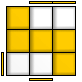R' F (R U R' U') y L' d R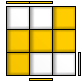L F' L' U' L U y' R d' L'